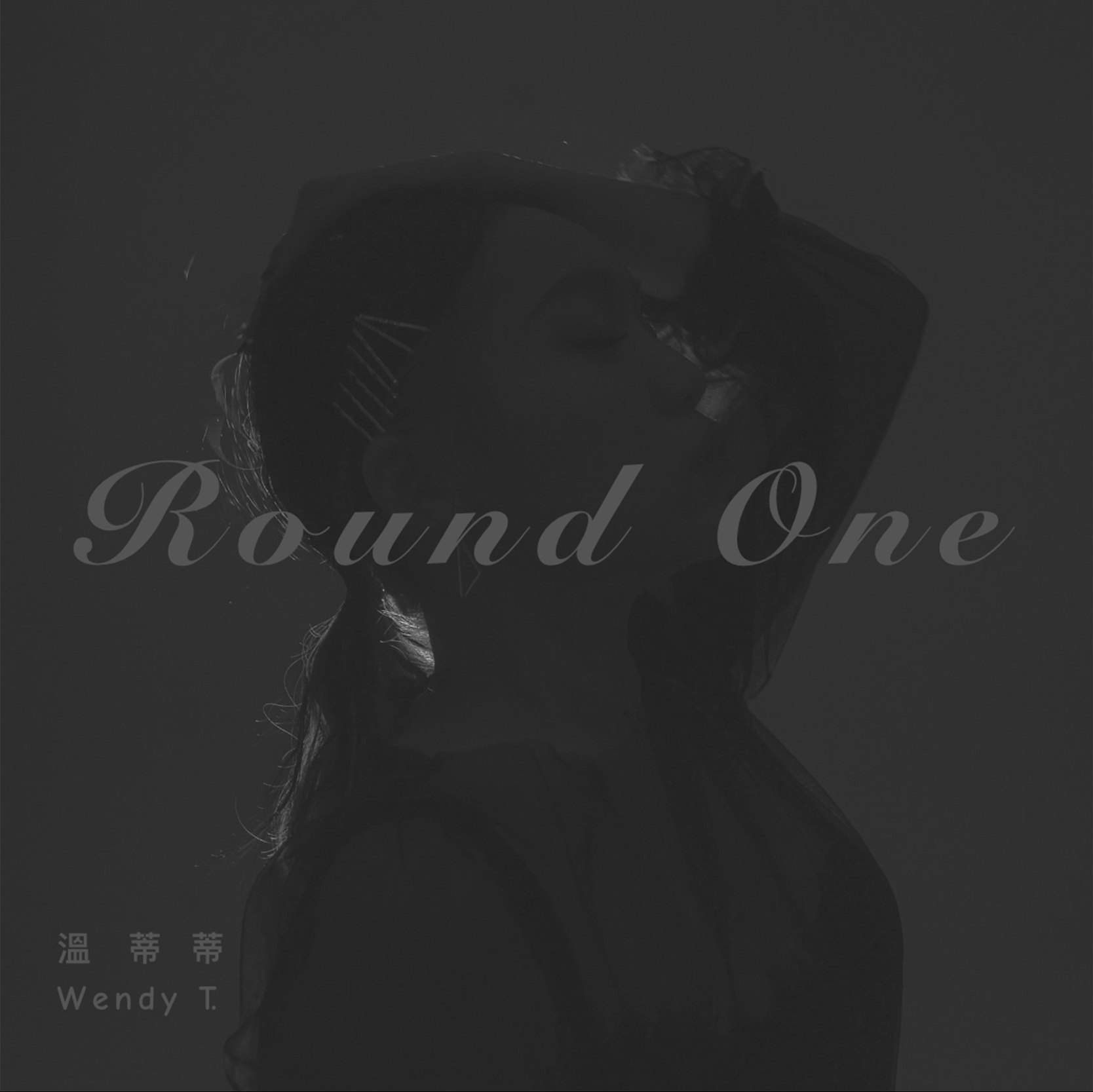## 相信你也會喜歡

• #### 暈Love

##### 田亞寧# Round One

### R&B / Soul ・ Round One## 介紹

M V請點➡️ https://youtu.be/Kzf8Bil-7oY

## 歌詞

So 燈不要全關 調成微暗

Round one Round one

Round one Round one

Before the round two

It’s just the Round one Round one

So 一層又一層地 束縛解開

Round one Round one

Round one Round one

Before the round two

It’s just the Round one Round one

Round one Round one

Round one Round one

Round one Round one
Round one Round one 來
Round one Round one
Round one Round one 來

Round one Round one

Round one Round one